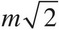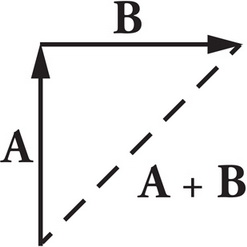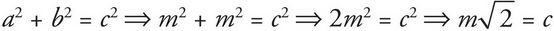# AP Physics 1 Question 181: Answer and Explanation

### Test Information

Question: 181

1. Two vectors, A and B, have the same magnitude, m, but vector A points north whereas vector B points east. What is the sum, A + B ?

• A. m, northeast
• B.northeast
• C.northwest
• D. 2m, northwest

Explanation:

B

To add the vectors, draw the first vector and then from the end of the first draw the second. The resultant vector is from the beginning of the first vector to the end of the second:The direction of the resultant vector is therefore northeast, eliminating (C) and (D). Since vectors A and B are perpendicular to each other and equal in magnitude, the magnitude of the resultant vector can be found using the Pythagorean Theorem:This makes (B) correct.